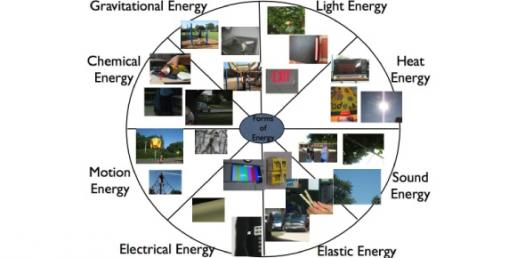# Forms Of Energy

17 Questions | Total Attempts: 2424SettingsThis is a pretest to test students on their previous knowledge on the forms of energy: kinetic, potential,work, force, inertia, etc.

Related Topics
• 1.
Something that makes an object start moving, stop moving, speed up, or slow down is a(n)
• A.

• B.

Position

• C.

Force

• D.

Effort

• 2.
The force that works against motion is
• A.

Friction

• B.

Newton

• C.

Direction

• D.

Acceleration

• 3.
The tendency of an object to stay in motion or at rest until a force acts upon it is
• A.

Frame of reference

• B.

Movement

• C.

Relation

• D.

Inertia

• 4.
A material that doesn't transfer heat very well is called a(n)
• A.

Applicator

• B.

Protector

• C.

Insulator

• D.

Conveyor

• 5.
A material that transfers heat well is known as a(n)
• A.

Conductor

• B.

Insulator

• C.

Producer

• D.

Inventor

• 6.
What is the major source of light energy?
• A.

Food cooking

• B.

The sun

• C.

Gravity

• D.

Electricity

• 7.
What kind of energy is found in our food?
• A.

Chemical energy

• B.

• C.

Thermal energy

• D.

Electrical energy

• 8.
What kind of energy does a stove give off that causes food to cook?
• A.

Chemical energy

• B.

Thermal energy

• C.

Electrical energy

• D.

Light energy

• 9.
What kind of energy comes fromt he motion of charged particles?
• A.

Thermal energy

• B.

Chemical energy

• C.

Electrical energy

• D.

Mechanical energy

• 10.
An object that has energy because of its position is said to have
• A.

Thermal energy

• B.

Kinetic energy

• C.

Potential energy

• D.

Force

• 11.
An example of potential energy is
• A.

A ball rolling down a hill

• B.

A ferris wheel

• C.

A rock sitting on top of a hill

• D.

An apple falling from a tree

• 12.
An example of kinetic energy is
• A.

A rock on top of a hill

• B.

Energy stored in a battery

• C.

A rolling bowling ball

• D.

Energy from stored food

• 13.
What is needed for a force to be applied to an object?
• A.

Friction

• B.

Force

• C.

Energy

• D.

Heat

• 14.
Kinetic energy is energy that is
• A.

Standing still

• B.

A type of gravity

• C.

Moving

• D.

Between friction and force

• 15.
Potential energy is energy that is
• A.

Standing still

• B.

A type of gravity

• C.

Moving

• D.

Between friction and force

• 16.
A swinging hammer is a form of
• A.

Force

• B.

Kinetic energy

• C.

Friction

• D.

Potential energy

• 17.
You can tell an object has moved when its ____________________ has changed.
• A.

Force

• B.

Position

• C.

Distance

• D.

Direction